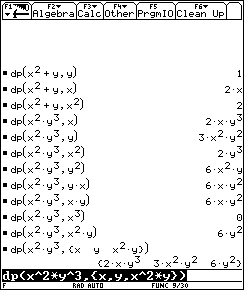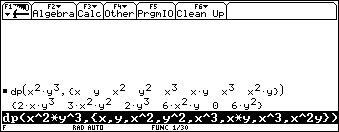## Texas Instruments TI-89 and TI-92 Plus Computer

Last page update: Sunday, November 21, 1999

 Partial Derivative (Single and Multivariable) - for the TI-89 and TI-92 Plus Version 1.0   (Monday, September 11, 1999) Download [ dp.89f  | dp.9xf ] (780 bytes) Description Single and mixed partial derivatives of one or higher orders. Screen shotsDocumentation Usage:  dp(Expr,VarOrList)  Expr: A continuous function.  VarOrList: The explicit variable(s) or a list of explicit variables. The order of the derivative is specified with an exponent for each variable(s). If VarOrList is a single variable or expression, a single expression will be returned; otherwise if VarOrList is a list of variables or expressions, a list of expressions will be returned. Note: The calculator's derivative function d() will return partial derivatives, but only to the first order. I have seen several other functions available on the Internet that will return partial derivatives of higher orders, but none of them will calculate mixed partial derivatives at higher orders as this one does (9/11/99).  Return value:  The partial derivative or a list of partial derivatives according to VarOrList.  Examples:  1. dp(x2y3 , x)  (fx(x,y))       2xy3 2. dp(x2y3 , {x , y})  (fx(x,y), fy(x,y))       {2xy3 , 3x2y2} 3. dp(x2y3 , x2)  (fxx(x,y))       2y3 4. dp(x2y3 , x*y)  (fxy(x,y))       6xy2 5. dp(x2y3 , x2*y2)  (fxxyy(x,y))       12y 6. dp(x2y3, {x2 , y2 , x2*y2})  (fxx(x,y) ,  fyy(x,y) ,  fxxyy(x,y))      {2y3 , 6x2y , 12y}